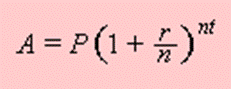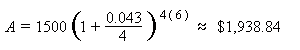# Compound Interest FormulaP = principal amount (the initial amount you borrow or deposit) r  = annual rate of interest (as a decimal) t  = number of years the amount is deposited or borrowed for. A = amount of money accumulated after n years, including interest. n  =  number of times the interest is compounded per year

Example:

 An amount of \$1,500.00 is deposited in a bank paying an annual interest rate of 4.3%, compounded quarterly. What is the balance after 6 years?

Solution:

 Using the compound interest formula, we have that P = 1500, r = 4.3/100 = 0.043, n = 4, t = 6. Therefore,So, the balance after 6 years is approximately \$1,938.84.